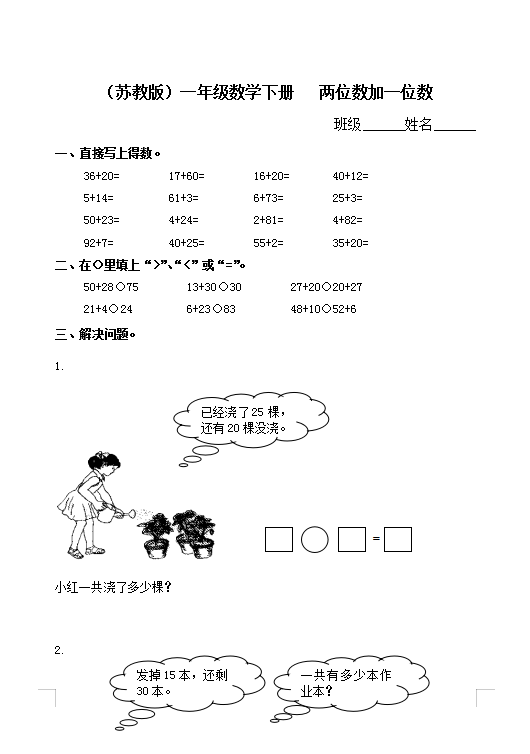（苏教版）小学一年级数学下册 两位数加一位数及答案 2（4页DOC文档）资料下载

（苏教版）小学一年级数学下册   两位数加一位数

36+20=        17+60=        16+20=       40+12=

5+14=         61+3=         6+73=        25+3=

50+23=        4+24=         2+81=        4+82=

92+7=         40+25=        55+2=        35+20=

50+28○75        13+30○30        27+20○20+27

21+4○24         6+23○83         48+10○52+6